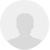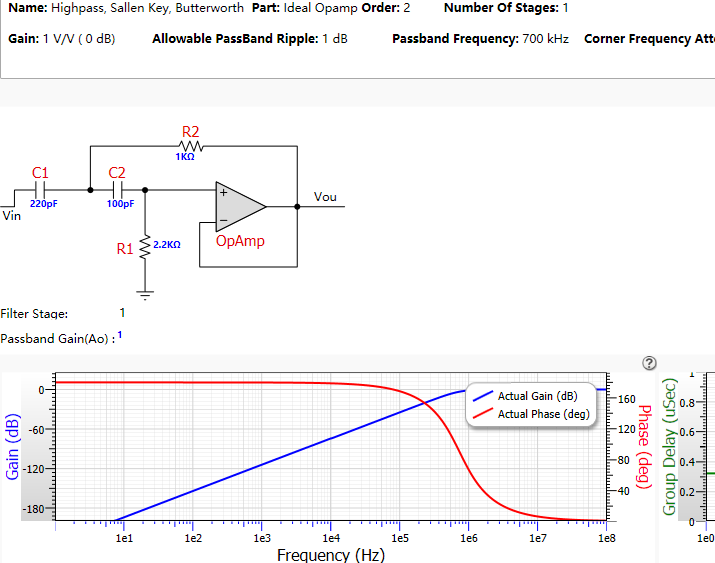# 【设计心得】FPGA之DS18B20

1
2026 2

```module DS18B02(
input							clk,
input							rst_n,

input	                  start,

//ds18b20 interface
inout							DQ,

input	[7:0]					rom_command,  //rom cpmmand data ,this only use cc
input	[7:0]             command,   //command data  this only use 44 and be

// input	[7:0]					data,   //write 8-bits data

output	reg					command_finish	,

output 						look_dq_in
);```

Start： 启动DS18B20发数据或者读数据。

Mode： 控制模式，1表示读数据，0表示写数据。

DQ： DS18B20的数据端口。

rom_command： 器件命令。

Command： 操作命令。

command_finish： 发送命令完成信号。

```reg DQ_reg;
assign DQ = enable_read ? 1'bz:DQ_reg;
assign look_dq_in = enable_read ? DQ:1'b0;```

```localparam idle_state ='d0;
localparam reset_state ='d1;
localparam write_rom_command_state ='d3;
localparam write_command_state ='d4;

```idle_state: begin
DQ_reg = 1'b1;
enable_read = 1'b0; //default DQ is output
delay_time_next  = 'd0;
bit_counter_next =  'd0;
ack_next			  =  'b1;
if(start)
state_next = reset_state;
end```

delay_time_next和之后的delay_time是用来对状态的持续时间进行计数的。因为要满足要求时序，所以每个状态要需要制定的时间才能进行跳转。

bit_counter_next和bit_counter用来指示传输的第几位。

ack_next 和ack用来保存读取到的存在脉冲的响应。

```reset_state: begin   //DQ output 0  600us
enable_read = 0;  //DQ is output
DQ_reg = 0;       //DQ output 0
if(delay_time >= 30000 - 1 )  //delay 600us
begin
delay_time_next = 'd0;
end
else
delay_time_next = delay_time + 1'b1;
end```

``` read_pulse_state: begin
enable_read = 1;  //DQ is input
DQ_reg = 1;
if(delay_time == 3500 - 1) //delay 70us
ack_next = DQ;
if(delay_time >= 25000 - 1 ) //delay 500us
begin
delay_time_next = 0;
//if receive pulse ack, write rom command, otherwise there is no ds18b20 is bus,go idle_state
if(ack == 0)
state_next = write_rom_command_state;
else
state_next = idle_state;
end
else
delay_time_next = delay_time + 1'b1;
end```

```write_rom_command_state: begin
enable_read = 0; //DQ is output
if(delay_time < 99)  //DQ output 0 keep  2us
DQ_reg = 0;
else if(delay_time < 4500 - 1) //delay 90us
if(rom_command[bit_counter] == 1)
DQ_reg = 1;
else
DQ_reg = 0;
else
DQ_reg = 1;
if(delay_time >= 5000 - 1) //delay 100us
begin
delay_time_next = 0;
if(bit_counter == 'd7)
begin
bit_counter_next = 'd0;
state_next = write_command_state;
end
else
bit_counter_next = bit_counter + 1'b1;
end
else
delay_time_next = delay_time + 1'b1;
end```

```write_command_state: begin
enable_read = 0; //DQ is output
if(delay_time < 99)  //DQ output 0 keep  2us
DQ_reg = 0;
else if(delay_time < 4500 - 1) //delay 90us
if(command[bit_counter] == 1)
DQ_reg = 1;
else
DQ_reg = 0;
else
DQ_reg = 1;
if(delay_time >= 5000 - 1) //delay 100us
begin
delay_time_next = 0;
if(bit_counter == 'd7)
begin
bit_counter_next = 'd0;
command_finish = 1'b1;
//if write, write finish ,go idle_state
if(mode == 0)
state_next = idle_state;
else
end
else
bit_counter_next = bit_counter + 1'b1;
end
else
delay_time_next = delay_time + 1'b1;
end```

``` read_state: begin
if(delay_time < 99)  //DQ output 0 keep  2us
begin
enable_read = 0; // DQ is output
DQ_reg = 0;           //DQ output 0
end
else if(delay_time < 4500 - 1) //delay 90us
begin
if(delay_time == 500 -1) //at 10us read DQdata
end
else
begin
enable_read = 0; // DQ is output
DQ_reg = 1;
end
if(delay_time >= 5000 - 1) //delay 100us
begin
delay_time_next = 0;
if(bit_counter == 'd15)
begin
bit_counter_next = 'd0;
state_next = idle_state;
end
else
bit_counter_next = bit_counter + 1'b1;
end
else
delay_time_next = delay_time + 1'b1;
end```

```module DS18B20_control(
input						clk,
input						rst_n,

input						start_ds18b20,

inout						DQ,

output					look_dq_in
);```

```localparam idle_state = 'd0;
localparam write_command_state = 'd1;
localparam wait_convert_state = 'd2;

```idle_state: begin
delay_time_next = 'd0;
if(start_ds18b20)
state_next = write_command_state;
end```

```write_command_state: begin
rom_command = 8'hcc;
command		= 8'h44;
start = 1;
if(command_finish == 1)
begin
start = 0;
state_next = wait_convert_state;
end
end```

DS18B20转换温度是需要时间的。不能说你发完命令后，就可以直接读取值。因为这个时候值还没有变化。所以需要等待时间。这里是等待1s。

```wait_convert_state: begin
if(delay_time >= 50_000_000 - 1) //delay 1s
//if(delay_time >= 50_0 - 1) //delay 1s
else
delay_time_next = delay_time + 1'b1;
end```

```read_temperature_state: begin
rom_command = 8'hcc;
command		= 8'hBE;
start = 1;
mode			= 'd1;
begin
start = 0;
state_next = idle_state;
end
end```

````timescale 1ns / 1ps
//////////////////////////////////////////////////////////////////////////////////
// Company:
// Engineer:
//
// Create Date:    18:09:53 11/27/2014
// Design Name:
// Module Name:    DS18B20_top
// Project Name:
// Target Devices:
// Tool versions:
// Description:
//
// Dependencies:
//
// Revision:
// Revision 0.01 - File Created
//
//////////////////////////////////////////////////////////////////////////////////
module DS18B20_top(
input					clk,
input					rst_n,

inout					DS18B20,

output	[7:0]    LED,

output				look_dq_in
);

reg start_ds18b20;

localparam  idle_state = 'd0;

reg state;
always@(posedge clk or negedge rst_n) begin
if(!rst_n)
begin
state <= idle_state;
start_ds18b20 <= 1'b0;
end
else
case(state)
idle_state:
begin
state <= idle_state;
start_ds18b20 <= 1'b0;
end
else
start_ds18b20 <= 1'b1;
end
endcase
end

DS18B20_control DS18B20_control_1(
.clk(clk),
.rst_n(rst_n),
.start_ds18b20(start_ds18b20),
.DQ(DS18B20),
.look_dq_in(look_dq_in)
);

endmodule```

1.dgddwh 2#

楼主，我只想让DS18B20温度达到一定值之后传输信号就行，请问我该从哪里修改

2.wfcnt 1#

***此内容已隐藏，只有楼主可见***